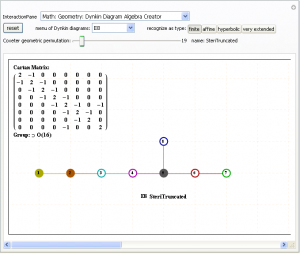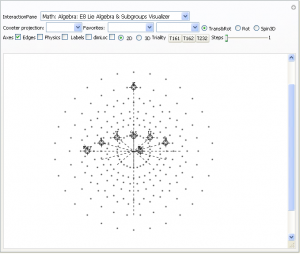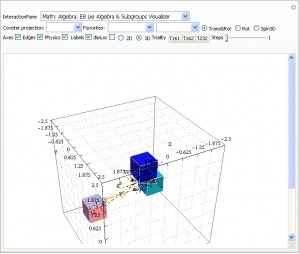# ToE=GR+SM

• Time is Octonion (1+I7D)
• Charge is Quaternion (1+I3D)
• Space is Complex- Real and Imaginary (1+I1D)*3
• (Higgs) Mass is Space/Real Time=Sqrt(Sqrt(2)hBar*l)=124.443…GeV
• 11D M-Theory collapses to Space+Time=4D+I7D

More detail to follow if you’re interested or have trouble dividing Sedenions 😉

# E8 to 4D (3D+T)

E8 8D to 4D (3D+T) Animation

Shown in this animation are the 240 vertices of E8 with shape, size, and color assigned based on theoretical physics of an extended Standard Model (eSM). It is made up of three sets of 120 frames, each with a different algorithm for calculating perspective and orthogonal, rotational and translational 8D flight paths. It is interesting to note that it is the 8D camera that is moving through 8D space and the vertices remain in their same 8D position.

The 30 blue triangles represent E8 triality relationships using an 8D rotation matrix based on 2Pi/3 (or 120 degrees). Each vertex in a blue triangle is transformed into an adjacent one by the dot product with the matrix. A second transformation transforms it to the next, while the third recovers the original vertex.

The 28 red and green triangles are created from a subset of the 6720 (shortest) edges of 8D norm’d length Sqrt(2). These are filtered to represent the particle sums (linked by a red line) for a common (clicked) vertex (linked by 2 green lines). It is interesting to note that all sums for a given vertex are only found in adjacent vertices.

Higher definition (2 sets 60 frames each):

# A Free Interactive Visual ToE Demonstration

This free Mathematica Computable Document Format (.CDF) demonstration takes you on an integrated visual journey from the abstract elements of geometry, algebra, particle and nuclear physics, and on to the atomic elements of chemistry. http://theoryofeverything.org/TOE/JGM/ToE_Demonstration.cdf (0.5Mb)
It requires the free Mathematica CDF plugin.# Higgs Mass Prediction of 124.443 GeV (a public retrodiction)

As shown in a previous post here and here, I introduced a prediction of a new 148 GeV particle that seemed to be evidenced by FermiLab’s CDF experiment. Unfortunately, the D0 experiment failed to confirm it. Initially I hesitated to make a public retrodiction (or post-diction) about the latest LHC findings of the Higgs at 124 Gev (until better confirmation).

I would like to make public some earlier work that produced eq. 18 148 GeV Higgs mass prediction. The issue is around the inclusion of the factor of 2 before the Planck constant. Initially, I anticipated that no factor would be needed, but that put the particle mass at ~98 GeV, below the exclusion range from LEP. I had entertained the idea of using an Sqrt(2) term putting it at ~124 GeV, but due to aesthetic considerations I opted for using a factor of 2. Please see the modified calculation highlighted below.

Interestingly, it seems the LHC has found that my earlier (albeit superceded) choice may have been the right one! But, time will tell…

# Ho-Mg-Zn QuasiCrystal Electron Diffraction with E8 5Cube Projection

This is an image of the electron diffraction pattern of an icosahedral Zn-Mg-Ho quasicrystal with an overlay of a 5-Cube projection from the 240 vertices of the split real even E8 Lie Group.

The basis vectors for the E8 projection are shown (1:1 with the ring of gray vertices with the last 3 of 8 dimensions 0).

There are 2480 overlapping edge lines from the 240 E8 vertices. They have norm’d unit length calculated from the 5 non-zero projected dimensions of E8. Of these, 32 inner vertices and 80 edges belong to the 5D 5-Cube (Penteract) proper. Edges are shown with colors assigned based on origin vertex distance from the outer perimeter.

The vertex colors of the 5-Cube projection represent E8 vertex overlaps. These are:
InView vertices={color{overlap,count},…}Total
{LightGreen{1,20},Pink{5,20},Gray{10,10},Orange{20,1}}51
All vertices={color{overlap,count},…}Total
{LightGreen{1,20},Pink{5,100},Gray{10,100},Orange{20,20}}240

A related projection of a 6D 6-Cube (Hexeract) into a perspective 3D object using the Golden Ratio [Phi]. This particular projection is used to understand the structure of QuasiCrystals. The specific basis vectors are:
x = {1, [Phi], 0, -1, [Phi], 0}
y = {[Phi], 0, 1, [Phi], 0, -1}
z = {0, 1, [Phi], 0, -1, [Phi]}
There are 64 vertices and 192 unit length edges forming pentagonal symmetry along specific axis (as well as hexagonal symmetries on other axis).